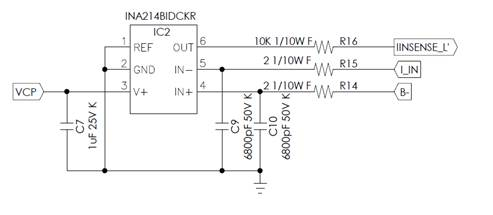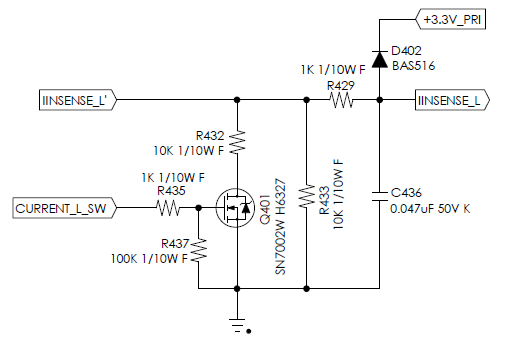If you have a related question, please click the "Ask a related question" button in the top right corner. The newly created question will be automatically linked to this question.

• Resolved

# INA214: INA214 Gain Error Factor Related to External Series Resistance

Part Number: INA214

Dear Team,

When the customer using INA214 to sense the input current at the input, they find that the same value of input current(different input voltage), OPA amplified value (pin6) is different.
For example, Rsense = 2 mohm, input voltage 40~72V, adjust the load so that the input current is about the same 5.7A
40V, 5.7A → 594 mV
48V, 5.7A → 616 mV
60V, 5.7A → 630 mV
72V, 5.7A → 652 mV
It is suspected that the input current is too dirty, causing the amplified voltage error.Subsequent adjustments the R14 & R15 resistance value from 2 ohms to 1 kohm or so, they found that the above problems can be improved (filter more strong);
But the whole gain drops from 100 to around 50, I think it should be related to gain error factor.

1. What is the side effect if we change R14 R15 so large, will there be other effects excepts smaller Gain's & slower response?

2.Why datasheet suggest "External Series Resistance" should smaller than 10 ohm?

3.what will you suggest to solve this issue? Change which components or change to which devices?

BR

Kevin

• Hi Kevin,

section 7.4.1 explains very well, why the filtering resistances must be kept to 10R. Don't overlook the box labelled "bias" in figure 24. This biasing network is what is causing a mismatch of input bias currents. So, increasing R14 and R15 to up to 1k is no option at all!

The 2mR shunt is sitting where? At the input voltage (high side) or at GND (low side)? What is the common mode input voltage? 5.7A x 2mR?

You wrote that you "adjust the load so that the input current is about the same 5.7A". How precise is "about"? Could this be the reason for the different output voltages?

What is the differential input voltage dropping across the shunt during your measurements? Can you measure it with a DVM (digital volt meter)?

Is there any ripple on the input voltage? Can you show a scope plot of the input voltages?

What is the purpose of R16? Why do you use such a high resistance there?

Kai
• In reply to kai klaas69:

Hi Kai,

Ok, I will keep the R14 & R15  as  2ohm.

The 2mR is sitting at GND (low side).  I  really  use DVM to measure  the shunt  R  cross voltage to make sure  "adjust the load so that the input current is about the same 5.7A", so I think there is no problem at precision.

R16  10kohm is use to  adjust output voltage for MCU ADC, shown as illustration. Does here has any problem ?Actually, I find the solution to solve this issue.

I adjust the C9 & C10 from 6800pF to 0.22uF, there has great improvement on this issue.

Hi Edward,

yes, increasing C9 and C10 is a very good idea, if the input signals are noisy. You could even add ferrite beads, as shown in section 7.4.5 of datasheet, if the input signals carry HF noise.

Ah ok, R16 is part of a resistive voltage divder. That makes sense now. Yes, you can do that.

Kai
• In reply to kai klaas69:

Dear Kai,

Thanks for the help, we can close this thread!

BR

Kevin